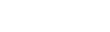# Understand how Cosine similarity works and build a simple web-based tool to check if two texts are similarEyuel Berga Woldemichael

4 months ago | 3 min read
FollowIn this article, we are going to build a text similarity checker using Cosine Similarity in JavaScript and HTML.

# What is cosine similarity?

Cosine similarity measures the similarity of two vectors. It measures the cosine of the angle between two vectors projected in a multi-dimensional space. We can use this metric to determine how similar two documents are irrespective of their size.

``cos(a,b) = a . b / ||a|| ||b||``

The cosine similarity between two texts can be found by using the above formula on the vector representation of each of the text’s word counts. We then can determine how similar those two texts are, in terms of word counts.

# Implementation

We will code all functions we need in JavaScript and we’ll also use HTML and some styling from MaterializeCSS to make a pleasant UI.

The first thing we need to do is map words in a text to their frequency count. To do that we just split the text into words loop through each word and count how many times the word appears in the text.

``function wordCountMap(str){    let words = str.split(' ');    let wordCount = {};    words.forEach((w)=>{        wordCount[w] = (wordCount[w] || 0) +1;    });return wordCount;}``

This is what the above function does, and at the end, it returns an object with mapping between a word and its frequency.

Next, we need to make a dictionary of all the words that are present in both the texts we are checking for similarity. We will then use our dictionary to make a vector representation of the word counts. To simplify the dictionary-making process, we add a function to extract the words from our word-frequency mapping and add it to our dictionary.

``function addWordsToDictionary(wordCountmap, dict){    for(let key in wordCountmap){        dict[key] = true;    }}``

Now we can change our word count map into a vector using our dictionary. The dimensions of our vectors will depend on the number of words we have in our dictionary.

``function wordMapToVector(map,dict){    let wordCountVector = [];    for (let term in dict){        wordCountVector.push(map[term] || 0);    }    return wordCountVector;}``

Now that we have the function to change text strings into vectors, we can start working on calculating their cosine similarity. As you recall from before, cosine similarity is the dot products of the two vectors divided by the product of their magnitude. We add three more functions to calculate the cosine similarity.

``function dotProduct(vecA, vecB){    let product = 0;    for(let i=0;i<vecA.length;i++){        product += vecA[i] * vecB[i];    }    return product;}function magnitude(vec){    let sum = 0;    for (let i = 0;i<vec.length;i++){        sum += vec[i] * vec[i];    }    return Math.sqrt(sum);}function cosineSimilarity(vecA,vecB){    return dotProduct(vecA,vecB)/ (magnitude(vecA) * magnitude(vecB));}``

Now we have everything we need, but let's make our life easier by adding a function that takes two strings rather than vectors.

``function textCosineSimilarity(txtA,txtB){    const wordCountA = wordCountMap(txtA);    const wordCountB = wordCountMap(txtB);    let dict = {};    addWordsToDictionary(wordCountA,dict);    addWordsToDictionary(wordCountB,dict);    const vectorA = wordMapToVector(wordCountA,dict);    const vectorB = wordMapToVector(wordCountB,dict);    return cosineSimilarity(vectorA, vectorB);}``

Okay, let's add a simple UI. We will use HTML and style it using MaterializeCSS. It looks like this:

We just need to add two more functions to display similarity results to our web page:

``function getSimilarityScore(val){    return Math.round(val * 100)}function checkSimilarity(){    const text1 = \$('#text1').val();    const text2 = \$('#text2').val();    const similarity = getSimilarityScore(textCosineSimilarity(text1,text2));    \$("#similarity").text(similarity+"%");}``

The getSimilarityScore function is just for rounding out the results and changing them into percentages, to make them more understandable. The last function is called when the Compare button is clicked and it manipulates the DOM using jQuery to display the result.

## Let's test it out

And there you have it, a simply made text similarity checker using cosine similarity metric… you can find the source code for this project here

UpvoteCreated by

Eyuel Berga Woldemichael

Follow

Software Developer

I am a Software Developer currently based in Addis Ababa, Ethiopia. I am passionate about Web and Mobile Development.Post

Upvote

Downvote

Comment

Bookmark

Share

Related Articles